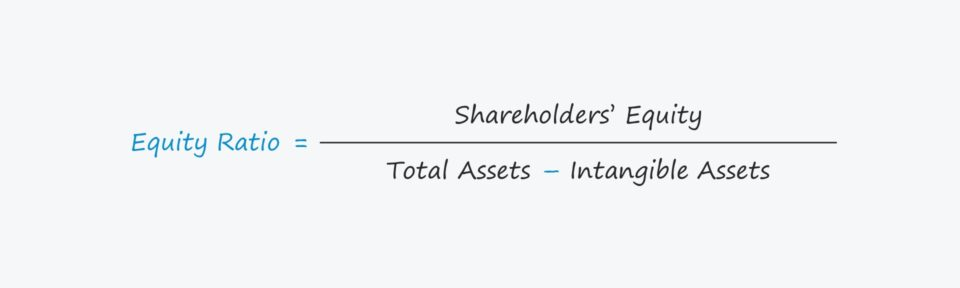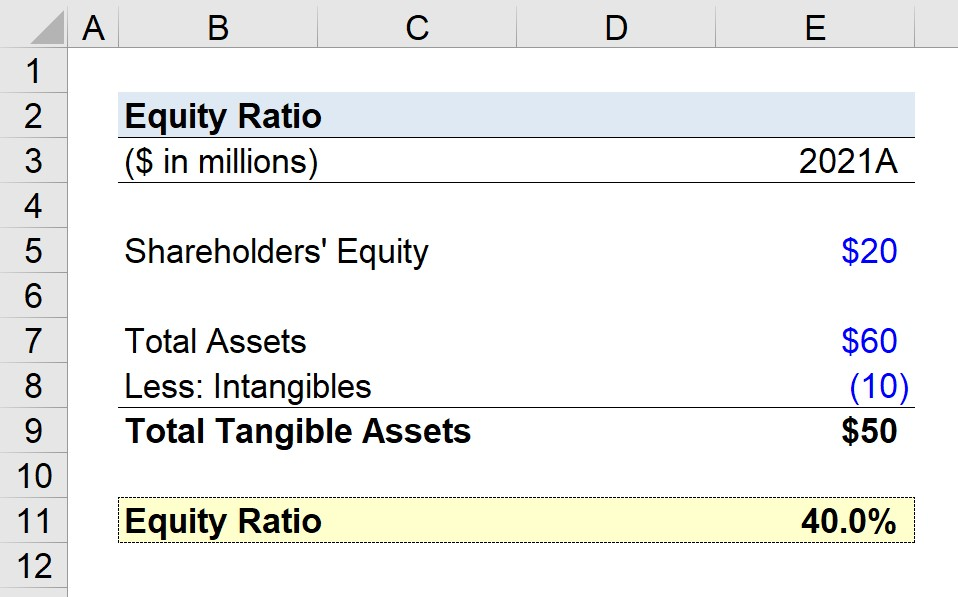Welcome to Wall Street Prep! Use code at checkout for 15% off.# Equity Ratio

Guide to Understanding the Equity Ratio## How to Calculate the Equity Ratio?

The equity ratio calculates the proportion of a company’s total assets financed using capital provided by shareholders.

The equity ratio, or “proprietary ratio”, is used to determine the contribution of shareholders to fund a company’s resources, i.e. the assets belonging to the company.

The purpose of the equity ratio is to estimate the proportion of a company’s assets funded by proprietors, i.e. the shareholders.

To calculate the equity ratio, there are three steps:

In practice, the proprietary ratio tends to be a reliable indicator of financial stability, as it provides insights into a company’s current capitalization (and how operations and capital expenditures are financed).

Of course, the ratio is inadequate to understand the fundamentals of a company and should be evaluated in conjunction with other metrics.

Still, the importance of the capital structure cannot be overstated, especially considering how practically all financially sound companies with a long-standing track record have sustainable capital structures well-aligned with their financial profiles.

In contrast, a capital structure that is unmanageable – i.e. the debt burden outweighs the company’s free cash flows (FCFs) – is one of the most common catalysts for corporate restructuring or causing a company to file for bankruptcy protection.

While the ratio cannot determine the optimal capital structure of a company, it can bring attention to an unsustainable reliance on debt financing which may soon lead to default (and potentially liquidation).

## Equity Ratio Formula

The formula for calculating the equity ratio is as follows.

Equity Ratio = Shareholders’ Equity ÷ (Total Assets Intangible Assets)

The ratio is expressed in a percentage, so the resulting figure must then be multiplied by 100.

The assets belonging to a company were somehow funded, i.e. either from equity or liabilities, the two primary funding sources:

1. Equity: Consists of items such as paid-in capital, additional paid-in capital (APIC), and retained earnings
2. Liabilities: Primarily refers to debt instruments in the context of funding, e.g. senior secured debt and bonds.

Intangible assets such as goodwill are normally excluded from the ratio, as reflected in the formula.

## What is a Good Equity Ratio?

The guidelines for what constitutes a “good” proprietary ratio are industry-specific and are also affected by the company’s fundamentals.

Still, as a general rule of thumb, most companies aim for an equity ratio of around 50%.

Companies with ratios ranging around 50% to 80% tend to be considered “conservative”, while those with ratios between 20% and 40% are considered “leveraged”.

• High Ratio → The higher the ratio, the less credit risk there is for the company, as the company does not rely much on creditors, e.g. commercial bank lenders and institutional debt lenders.
• Low Ratio → On the other hand, a lower ratio signifies that the company is dependent on creditors. If the percentage of debt far exceeds the equity interests, the company may be at risk of insolvency.

If the company faced unexpected headwinds and subsequently underperformed, it could soon be in trouble unless it can obtain more external financing, which can be difficult if the near-term outlook on the economy is negative, and the conditions of the credit markets are also bleak.

However, it is also untrue that the higher the ratio, the better off the company, as a near 100% equity ratio is considered “over-conservative”.” In such a case, companies are missing out on the benefits of using leverage, such as the interest tax shield and debt financing being a “cheaper” source of capital.

## Equity Ratio Calculator

We’ll now move to a modeling exercise, which you can access by filling out the form below.Submitting...

## Equity Ratio Calculation Example

Suppose we’re tasked with calculating the equity ratio for a company in its latest fiscal year, 2021.

At the end of 2021, the company reported the following carrying values on its balance sheet.

• Shareholders’ Equity = \$20 million
• Total Assets = \$60 million
• Intangibles = \$10 million

Since we’re working to first calculate the total tangible assets metric, we’ll subtract the \$10 million in intangibles from the \$60 million in total assets, which comes out to \$50 million.

• Total Tangible Assets = \$60 million – \$10 million = \$50 million

With all the necessary assumptions, we can simply divide our shareholders’ equity assumption by the total tangible assets to achieve an equity ratio of 40%.

• Equity Ratio = \$20 million ÷ \$50 million = 0.40, or 40%

The 40% equity ratio implies that shareholders contributed 40% of the capital used to fund day-to-day operations and capital expenditures, with creditors contributing the remaining 60%.Step-by-Step Online Course

### Everything You Need To Master Financial Modeling

Enroll in The Premium Package: Learn Financial Statement Modeling, DCF, M&A, LBO and Comps. The same training program used at top investment banks.